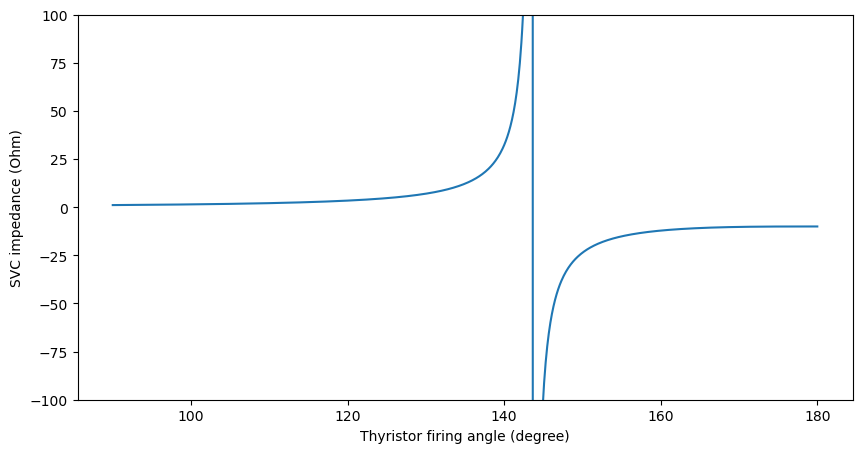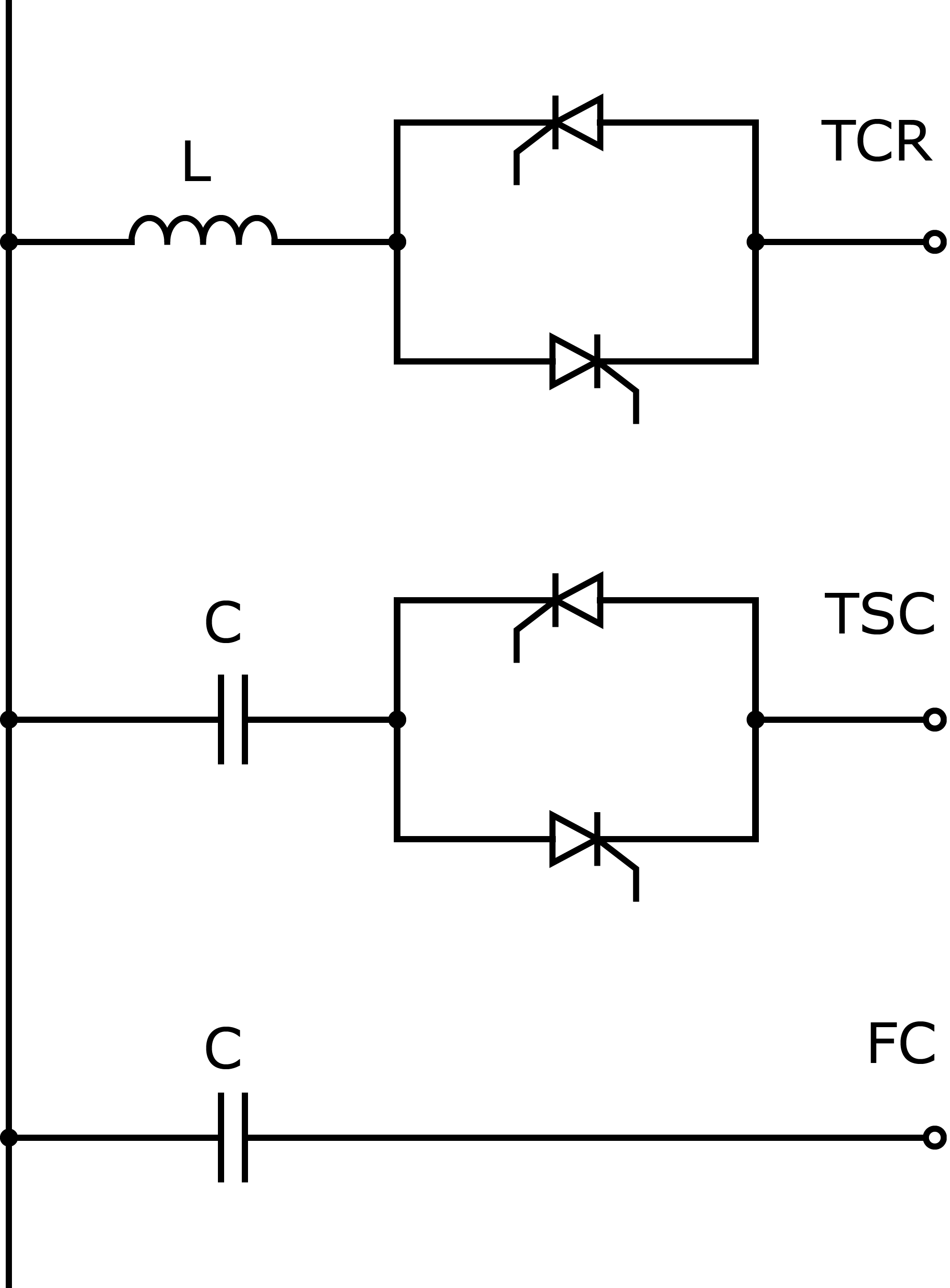# Static Var Compensator (SVC)

We implement the FACTS devices based on the following source:

A. Panosyan, “Modeling of advanced power transmission system controllers”, Ph.D. dissertation, Gottfried Wilhelm Leibniz Universität Hannover, 2010.

The element SVC is a shunt-connected thyristor-based power electronic device. It regulates the voltage magnitude at the connected bus by adjusting the shunt impedance value. The shunt impedance only has the reactance component (no resistive part). The impedance can be capacitive or inductive, because the device consists of a fixed capacitor in a parallel circuit to the reactor that is regulated by a pair of antiparallel thyristors. The thyristor firing angle regulates the total impedance of the element.

The active range of the device is presented in the figure below:It can be seen that the device has a resonance region between the inductive (positive) and the capacitive (negative) impedance characteristics. In real application, the operation of the device in the resonance region is prohibited by defining the inadmissible range for the thyristor firing angle around the resonance point. We did not implement this in our software - this should be considered for future work.

The device operates in the value range of the thyristor firing angle between 90° and 180°. Any results for the thyristor firing angle outside of this range are invalid (we have not observed a converged result with values outside of the valid range in the test cases). Even though we include the values of min_angle_degree and max_angle_degree in the create function, they are not considered during the power flow calculation. For future work, the functionality similar to “enforce_q_lims” should be implemented.

We demonstrate the use-case of this device in the pandapower tutorial: FACTS.

## Create Function

pandapower.create.create_svc(net, bus, x_l_ohm, x_cvar_ohm, set_vm_pu, thyristor_firing_angle_degree, name=None, controllable=True, in_service=True, index=None, min_angle_degree=90, max_angle_degree=180, **kwargs)

Creates an SVC element - a shunt element with adjustable impedance used to control the voltage at the connected bus

Does not work if connected to “PV” bus (gen bus, ext_grid bus)

min_angle_degree, max_angle_degree are placeholders (ignored in the Newton-Raphson power flow at the moment).

INPUT:

net (pandapowerNet) - The pandapower network in which the element is created

bus (int) - connection bus of the svc

x_l_ohm (float) - inductive reactance of the reactor component of svc

x_cvar_ohm (float) - capacitive reactance of the fixed capacitor component of svc

set_vm_pu (float) - set-point for the bus voltage magnitude at the connection bus

thyristor_firing_angle_degree (float) - the value of thyristor firing angle of svc (is used directly if

controllable==False, otherwise is the starting point in the Newton-Raphson calculation)

OPTIONAL:

name (list of strs, None) - element name

controllable (bool, True) - whether the element is considered as actively controlling or

as a fixed shunt impedance

in_service (bool, True) - True for in_service or False for out of service

index (int, None) - Force a specified ID if it is available. If None, the

index one higher than the highest already existing index is selected.

min_angle_degree (float, 90) - minimum value of the thyristor_firing_angle_degree

max_angle_degree (float, 180) - maximum value of the thyristor_firing_angle_degree

OUTPUT:

index (int) - The unique ID of the created svc

## Input Parameters

net.svc

 Parameter Datatype Value Range Explanation name string name of the SVC bus* integer index of bus where the SVC is connected x_l_ohm* float $$\geq$$ 0 impedance of the reactor component of SVC x_cvar_ohm* float $$\leq$$ 0 impedance of the fixed capacitor component of SVC set_vm_pu* float set-point for the bus voltage magnitude at the connection bus thyristor_firing_angle_degree* float :math:90 leq 180 the value of thyristor firing angle of SVC controllable* boolean True / False whether the element is considered as actively controlling or as a fixed shunt impedance in_service* boolean True / False specifies if the SVC is in service. min_angle_degree float $$\geq$$ 90 minimum value of the thyristor_firing_angle_degree max_angle_degree float $$\leq$$ 180 maximum value of the thyristor_firing_angle_degree

*necessary for executing a power flow calculation.

## Electric ModelThe shunt impedance $$X_{SVC}$$ of the SVC element is calculated according to the following equation:

\begin{align*} X_{SVC} &= \frac{\pi X_L}{2 (\pi - \alpha) + \sin{(2\alpha)} + \frac{\pi X_L}{X_{Cvar}}} \end{align*}

The term $$X_L$$ stands for the reactance of the reactor (x_l_ohm) and the term $$X_{Cvar}$$ stands for the total capacitance (x_cvar_ohm). The thyristor firing angle $$\alpha$$ is the state variable that on the one hand defines the impedance of the element, and at the same time is the result of the Newton-Raphson calculation.

The reactive power consumption of the SVC element is calculated with:

\begin{align*} Q_{SVC} = \frac{V^2}{X_{SVC}} \end{align*}

Where V is the complex voltage observed at the connection bus of the SVC element. The reference values for the per unit system as defined in Unit Systems and Conventions.

## Result Parameters

net.res_svc

 Parameter Datatype Explanation thyristor_firing_angle_degree* float the resulting value of thyristor firing angle of svc [degree] x_ohm float resulting value of the shunt impedance of svc [Ohm] q_mvar float shunt reactive power consumption of svc [MVAr] vm_pu float voltage magnitude at svc bus [pu] va_degree float voltage angle at svc bus [degree]